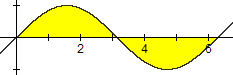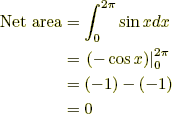# area under a curve

Area under a Curve

The area between the graph of y = f(x) and the x-axis is given by the definite integral below. This formula gives a positive result for a graph above the x-axis, and a negative result for a graph below the x-axis.

Note: If the graph of y = f(x) is partly above and partly below the x-axis, the formula given below generates the net area. That is, the area above the axis minus the area below the axis.

 Formula: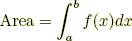Example 1: Find the area between y = 7 – x2 and the x-axis between the values x = –1 and x = 2.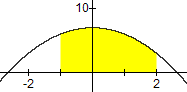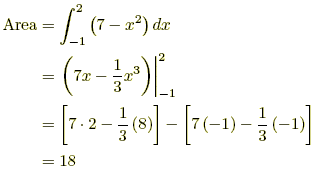Example 2: Find the net area between y = sin x and the x-axis between the values x = 0 and x = 2π.